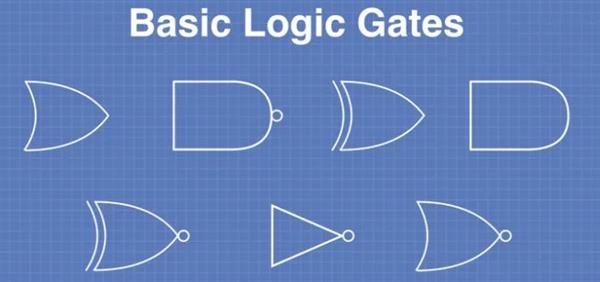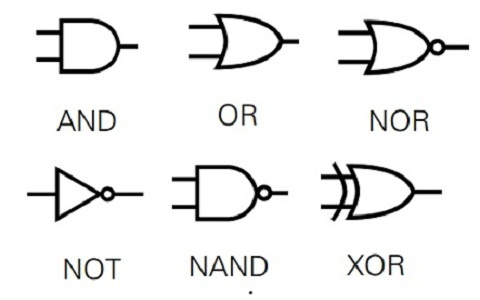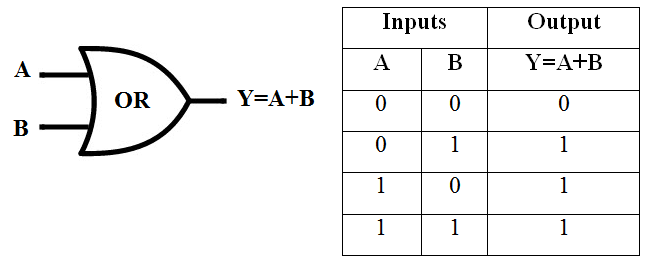Loading, please wait...# Basic Logic gates in details

Dec 22, 2021 Logic, gates, or, and, not, nand, xor, 3338 Views
There are often a lot of basic gates. These basic gates are used by microprocessors and microcontrollers. Basic gates form the foundations for all digital electronic components and devices, just as simple atoms can create molecules that in turn can make crickets, elephants or people.

## Introduction

The world we live in is digital. Our radios, televisions, and audio equipment are no longer analog devices, we all own computers and smartphones, and our appliances and even our light bulbs are now microcontroller-based.

Digital signals can only exist at two levels. This is in contrast to analog signals which can exist at infinite levels. To represent zeroes or ones, our digital devices use two voltage levels. These signals can be modified by basic logic gates.

There are often a lot of basic gates. These basic gates are used by microprocessors and microcontrollers. Basic gates form the foundations for all digital electronic components and devices, just as simple atoms can create molecules that in turn can make crickets, elephants, or people.

### It's the 21st Century, however!

One might assume that basic logic gates no longer have any use for designing new products, given the affordability of microcontrollers. This is false.

Both with and without microcontrollers, basic logic gates are still very popular. They can be used to create simple circuits and offer performance and cost advantages. They can be combined with MCU's and CPU's to create complex designs or simplify programming.

Even if your circuits don't use basic gates, you still have the opportunity to benefit from their knowledge. This will allow you to fix existing circuits, and also give you a better understanding about the inner workings of modern electronic components.

Let's get started!

## Basic GatesYou'll find devices with intriguing names such as "monostable multivibrators", or "edge-triggered flipflops" in a list of basic digital components. These devices are definitely "building blocks" in digital technology, but they aren't basic gates. They are actually made up of basic gates.

A "basic gateway" is a component that has one input and one output. All inputs and outgoings are digital.

There are three basic gates, plus seven basic logic gates.Based on the state of the input signals, the gate will set its output value to zero or one. The output condition is determined by the rules of boolean algebra.

### Truth Tables

A "truth table" is a great way to illustrate the relationship between input and output logic levels of a gate.

A truth table is a chart or table that lists all possible input combinations as well as the resulting output logic level. This is an example truth table:

 A B Y 0 0 0 0 1 1 1 0 1 1 1 0

The inputs to our gate have been labeled with "A" or "B", while the output is labeled with "Y".

• If both inputs to our gate have zero, the output will be zero.
• If A=0 and A=1, the output is one.
• If A=1 and A=0, the output is one.
• If both inputs equal one, then the output will be zero.

The truth table can be used to determine the logic of the gate. The truth table above is for an "Exclusive OR". This gate and six others will be examined right now.

### NOT Gate

The NOT gate is one of the most basic gates. It also has the lowest input. It is the first gate of the three elementary ones.This type of gate is also known as an "Inverter" and it is easy to see why. The NOT gate output is the opposite of its input. In other words, it transforms zeros into one and zeros into one.

It is also possible to see the boolean algebra description for the NOT gate. The line above the "Y", indicates that the output has been inverted.

Although it is quite simple, the NOT gate can be very useful. Inverting digital signals is an important requirement in designing logic circuits.

### AND Gate

The AND gate, which is the second of three elementary gates is shown below.The truth table shows that the AND gate outputs zero if all its inputs are at the same level.

Also, you'll notice that the AND operation symbol is a boolean symbol.

### OR Gate

The OR gate is the third of the three elementary gates, as illustrated below.When any inputs to the OR gate are set to 1, the output of that gate is one.

It can be confusing to use the boolean symbol as an OR operation, since it is the same symbol that is used in addition in standard mathematics. Boolean algebra 1 + 0 is indeed equal to 1, but 1 + 1 equals 1.

### Exclusive OR Gate

The four remaining gates are largely derivatives of the three previous ones.

Here is the Exclusive OR (or XOR gate). It is worth looking at the truth table as it best explains the operation.As you can see, the output of an Exclusive OR gate will be one if only one input has been set. The output will become zero if more than one input is set to one.

The boolean symbol Exclusive OR is the + sign (a boolean OR with a circle around).

### NAND Gate

A NAND Gate simply refers to an AND gate that has an inverted output.This operation is completely opposite to the AND gate. If all inputs are equal, the output will be one.

This is a common gate. I'll show you why in a moment.

### NOR Gate

A NOR gate, like the NAND gate is an OR gate with inverted output.A NOR gate can only output one if both inputs are zero.

The boolean formula to create a NOR gate uses lines to indicate an inversion.

### Exclusive NOR Gate

You've guessed it! An inverted Exclusive NOR gate is an exclusive OR gate!This is sometimes called an "XNOR" gate. If all inputs are equal, it will output a one. Any other combination will result in a zero output.

## Conclusion

Although basic logic gates are simple, you can put enough together to build almost anything. These logic gates can be used as a standalone device or to augment a microcontroller/microcomputer.

Understanding the operation of logic gates will help you gain more insight into the internal workings of electronic devices that shape our lives and surround us.

It's amazing to think that this all works by manipulating a lot of zeros, ones.